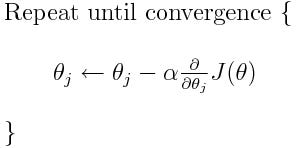# Why is Mean Squared Error used in Machine Learning?

## Various reasons for why the cost function uses Mean Squared Error

Posted by Vivek Verma on January 15, 2019

If you've ever tried doing machine learning, you probably saw that the cost function uses mean squared error instead of just measuring the distance. There are various reasons why this is done, but I just want to go over how gradient descent works before we talk about them.

So, let’s see how our cost function could look. Our goal is to obtain a local minimum. The way gradient descent works is by taking small steps in the negative direction of the slope. You can see how this works - the downhill direction is always the opposite direction of the slope.

Computing these steps depends on a constant, called the learning rate. We can iterate some number of times and keep doing these steps. Using partial derivatives, we can calculate the slope at each of the points and adjust accordingly. Let’s say alpha is our learning rate. Our equations for gradient descent would look like this.You can see how we subtract the derivative at each of the points. We can keep performing these steps, and if we have a good learning rate, we should converge to a local minimum. This brings me to the first reason why we use mean squared error- because it makes J differentiable.

Let’s say we have a function $y = |x|$. At $x=0$, y is not differentiable. Since we use derivatives in gradient descent, it is important that our cost function is differentiable for all x. When using mean square error, the estimator and variance become linear, which gives it much nicer properties.

When I say linear, I don’t mean a function that is expressed by $f(x) = mx +b$. In linear algebra, a linear function is something that satisfies the following properties - It preserves addition and scalar multiplication. This basically means that $f(x + y) = f(x) + f(y)$ and $f(a * x) = a * f(x)$.

Another important thing is that the square error is the inner product between the difference of the vectors and itself. An inner product is similar to the dot product, where you project a vector along another one and add the vectors together, but there are a few rules:

1. The inner product has conjugate symmetry:
$\langle {x, y} \rangle = \bar{\langle {y, x} \rangle}$
2. The inner product is linear in the first argument:
$\langle {ax, y} \rangle = a\langle{x,y}\rangle$
$\langle {x+z, y} \rangle = \langle{x,y}\rangle + \langle{z,y}\rangle$
3. The linear product has positive-definiteness:
$\langle {x,x}\rangle \geq 0$
$\langle {x,x}\rangle = 0 \Leftrightarrow x=0$
The euclidean distance between two vectors is the inner product between the difference between them and itself. The mean squared error can now be defined as an inner product.

So there you go! That’s why we use mean square error instead of absolute value. It’s mostly conveniences, which actually help quite a lot. Thanks for reading!